Courses

# Colligative Properties (Part-1) Chemistry Notes | EduRev

## Chemistry : Colligative Properties (Part-1) Chemistry Notes | EduRev

The document Colligative Properties (Part-1) Chemistry Notes | EduRev is a part of the Chemistry Course Physical Chemistry.
All you need of Chemistry at this link: Chemistry

1. Solution

In chemistry, a solution is a special type of homogeneous mixture composed of two or more substances. The term aqueous solution is when one of the solvents is water. In such a mixture, a solute is a substance dissolved in another substance, known as a solvent. The mixing process of a solution happens at a scale where the effects of chemical polarity are involved, resulting in interactions that are specific to solvation. The solution assumes the phase of the solvent when the solvent is the larger fraction of the mixture, as is commonly the case. The concentration of a solute in a solution is the mass of that solute expressed as a percentage of the mass of the whole solution.

2. Charactersticts

1. A solution is a homogeneous mixture of two or more substances.
2. The particles of solute in a solution cannot be seen by the naked eye.
3. A solution does not allow beams of light to scatter.
4. A solution is stable.
5. The solute from a solution cannot be separated by filtration (or mechanically).
6. It is co mposed of only one phase.

3. Types

Homogeneous means that the components of the mixture form a single phase. Heterogeneous means that the components of the mixture are of different phase. The properties of the mixture (such as concentration, temperature, and density) can be uniformly distributed through the vo lume but only in absence of diffusio n phenomena or after their completion. Usually, the substance present in the greatest amount is considered the solvent. Solvents can be gases, liquids or solids. One or more components present in the solution other than the solvent are called so lutes. The solut ion has the same physical state as the solvent.

A. Gaseous solutions

If the solvent is a gas, only gases are dissolved under a given set of condit ions.An example of a gaseous solut ion is air (oxygen and other gases disso lved in nitrogen). Since interact ions between mo lecules play almost no role, dilute gases form rather trivial solutions. In part of the literature, they are not even classified as solut ions, but addressed as mixtures.

B. Liquid solutions

If the solvent is a liquid, then almost all gases, liquids, and solids can be dissolved. Here are some examples:

1) Gas in liquid:

· Oxygen in water

· Carbon dioxide in water – a less simple example, because the solution is accompanied by a chemical reaction (formation of ions). Note also that the visible bubbles in carbonated water are not the disso lved gas, but only an effervescence of carbon dioxide that has co me out of so lut ion; the dissolved gas itself is not visible since it is dissolved on a molecular level.

2) Liquid in liquid:

· The mixing of two or more substances of the same chemistry but different concentrations to form a constant. (Homogenization of solutions)

· Alcoholic beverages are basically solutions of ethanol in water.

3) Solid in liquid:

· Sucrose (table sugar) in water

· Sodium chloride (NaCl) (table salt) or any other salt in water, which forms an electrolyte: When disso lving, salt dissociates into ions.

· Solutions in water are especially common, and are called aqueous solut ions.

· Non-aqueous solutions are when the liquid solvent involved is not water.

Counter examples are provided by liquid mixtures that are not homogeneous: colloids, suspensions, emulsions are not considered solutions.

Body fluids are examples for complex liquid solutions, containing many solutes. Many of these are electrolytes, since they contain solute ions, such as potassium. Furthermore, they contain solute molecules like sugar and urea. Oxygen and carbon dioxide are also essential components of blood chemistry, where significant changes in their concentrations may be a sign of severe illness or injury.

C. Solid solutions

If the solvent is a solid, then gases, liquids and solids can be dissolved.

1) Gas in solids:

· Hydrogen dissolves rather well in metals, especially in palladium; this is studied as a means of hydrogen storage.

2) Liquid in solid:

· Mercury in gold, forming an amalgam· Water in so lid salt or sugar, forming mo ist solids · Hexane in paraffin wax

3) Solid in solid:

· Steel, basically a solution of carbon atoms in a crystalline matrix o f iron atoms

· Alloys like bronze and many others

· Polymers containing plasticizers

4. Vapour Pressure of a Solution

Consider a pure liquid in a beaker is covered with a jar. Some molecules of the liquid on the surface evaporate and fill the vacant space available to them. The molecules in the vapour phase move randomly in the vacant space, during this motion, they strike the surface of the liquid and get condensed. This process of evaporation and condensation go on and anequilibrium is established between evaporation and condensation. The pressure exerted by vapours over the liquid surface at this equilibrium is called vapour pressure of the liquid.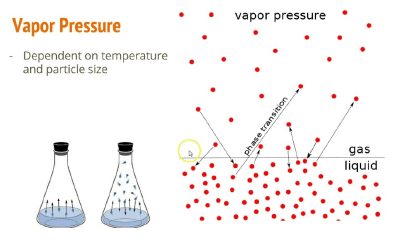If solution is non volatile solid or liquid the vapour pressure of solution is equal to partial vapour pressure of solvent in the solution and if the solute and if the solute is volatile solid or liquid, then a vapour pressure will be equal to the sum of partial vapour pressure of solute and that of solvent.

5. Raoult’s Law

This law is applied for a solution of liquid in liquids and can be stated as follows. “The partial vapour pressure of any component in the solution is directly proportional to its mole fraction”.

PA ∝ XA            where, PA = Partial vapour pressure of XA
PA = KXA         XA = Mole fraction of A in solution

For pure liquids XA = 1

Then K = P°A where P°A is the vapour pressure of component A is pure state

Hence, PA = XAA, similarly for component B, PB = XBB

6. Raoult’s law in combination with Dalton’s law of partial pressure

Assuming that vapours of a liquid is behaving like an ideal gas, then according to Dalton’s law of partial pressure the total pressure PT is given by

PT = PA + PB

= XAP0A + XBP0B

= XAP0A + (1-XA) P0B = Vapour pressure in terms of Mole fraction of Solvent.

= P0B + (P0A - P0B)XA

Example: The vapour pressure of ethanol and methanol are 44.5 mm and 88.7 mm Hg respectively. An ideal solution is formed at the same temperature by mixing 60 g of ethanol with 40 g of methanol. Calculate total vapour pressure of the solution.

Solution: Number of moles of ethanol = 60/40 = 1.5

Number of moles of methanol = 40/32 = 1.25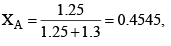XB 1-0.4545 = 0.545

Let A = CH3OH, B = C2H5OH

Total pressure of the solution

PT = XAA + XBA = 0.4545 × 88.7 + 0.545 × 44.5 = 40.31 + 24.27 = 64.58 mm Hg

7. Composition of the vapour

The composition of the liquid mixture and vapour that are in mutual equilibrium are not necessarily the same, the common sense suggest that the vapour pressure should be richer in the more volatile component. This expectation can be confirmed as follows:

Let the mole fract ion of A and B in vapour phase be YA and YB then from Dalton’s law,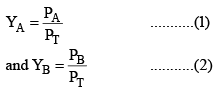Provided the mixture of vapours behaves as an ideal gas

Rewriting equation (1)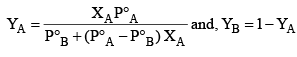Example: What is the composition of the vapour which is in equilibrium at 30°C with a benzene toluene solution with a mole fraction of benzene of 0.400?

(P°B = 119 torr and P°T = 37.0 torr)

Solution: Total pressure of the solution is given by

PT = XBB + XTP°T

= 0.4 × 119 + 0.6 × 37 = 47.6 + 22.2

= 69.8 torr

Applying Dalton’s law for mole fraction is vapour phase.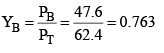YT = 1 – 0.763 = 0.237

8. Condensation of vapour of solution

When the vapours of solution (containing liquids A and B is) condensed, the composition of liquids A and B in the condensate remains same. Vapour over condensate can again recondens and the composition of A and B in condensate (2) remains same as it was in vapour phase over condensate (1).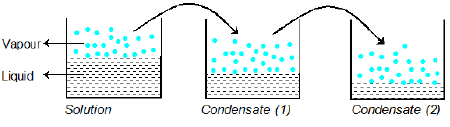Example: Liquid A and B form an ideal solution. The vapour pressure of A and B at 100°C are 300 and 100 mm Hg respectively. Suppose that vapour above solution is composed of 1 mole of A and 1 mole of B is collected and condensed. This condensate is then heated at 100°C and vapour are again condensed to form a liquid L. What is the mole fraction of A in the vapours of L?

Solution: Vapour pressure due to vapours above solution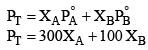It is given that in vapour phase each of A and B are one mole each hence each of them have mole fraction 0.5 in vapour phase.

After condensation of vapours.

In condensate (1)

X'A 0.5, X'B 0.5

P'T 0.5 × 300 0.5 × 100 150 + 50 = 200 mm

Mole fraction A and B in vapour phase of condensate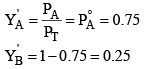When the vapours of the condensate (1) will again be vaporized in condensate (2) liquid L.

X"A = 0.75, X"B = 0.25, where X"A  and X"B are mole fraction of A and B in liquid L.

P"T = 300 × 0.75 + 100 × 0.25 = 225 + 25 = 250 mm and mole fraction of A in vapour phase of the condensate (2) is given by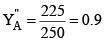9. Ideal solutions

The solutions which obey raoult’s law at all compositions of solute in solvent at all temperature are called ideal solution.

Ideal solution can be obtained by mixing two components with identical molecular size, structure and they should have almost same inter molecular attraction e.g., two liquids A and B form an ideal solution when A-A and B-B molecular attractions will be same and hence A-B molecular attraction will be almost same as A-A and B-B molecular attractions.

An ideal solution should have following characteristics

1. It should obey Raoult’s law i.e., PA = XAA and PA = XBB

2. ΔHmixing = 0, i.e. no heat should be absorbed or evolved during mixing.

3. ΔHmixing = 0, i.e. no expansio n of contraction on mixing.

Examples of Ideal solutions:

(i) Ethyl chloride and ethyl bro mide

(ii) n-hexane and n-heptane

(iii) CCl4 and SiCl4

The solution which deviate from ideal behaviour are called non ideal solution or real solutions and they do not obey Raoult’s law over entire range of composition. It has been found that on increasing dilution, a non ideal solution tend to be ideal.

10. Non ideal solutions

Many pairs of liquids are present in which there is no uniformity of attractive forces, i.e., the adhesive and cohesive forces of attraction are not uniform between the two liquids, so that they deviate from the Raoult's law applied only to ideal solutions.

For non ideal solutions,

(i)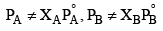i.e. they do not obey Raoult's law

(ii) ΔHmixing ≠ 0
(iii) ΔVmixing ≠ 0

Here, we have two cases:

Positive Deviation

When  (i) PA > XAA & PB > XBB
(ii) ΔHmix > 0,
(iii) ΔVmix > 0

When the cohesive forces between like molecules are greater than the adhesive forces between dissimilar molecules, the dissimilarities of polarity leads both components to escape solution more easily. Therefore, the vapor pressure is greater than expected from the Raoult's law, showing positive deviation. If the deviation is large, then the vapor pressure curve shows a maximum at a particular composition and form a positive azeotrope. Some mixtures in which this happens are (1) benzene and methanol, (2) carbon disulfide and acetone, and (3) chloroform and ethanol. When these pairs of components are mixed, the process is endothermic reaction as weaker intermolecular forces are formed so that ΔmixH is positive.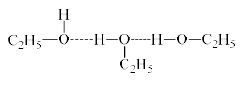Negative Deviation

(i) PA < XAA  & PB < XBB
(ii) ΔHmix < 0
(iii) ΔVmix < 0

If the vapor pressure of a mixture is lower than expected from Raoult's law, there is said to be a negative deviation.

Negative deviations from Raoult's law arise when the forces between the particles in the mixture are stronger than the mean of the forces between the particles in the pure liquids. This is evidence that the adhesive forces between different components are stronger than the average cohesive forces between like components. In consequence, each component is retained in the liquid phase by attractive forces that are stronger than in the pure liquid so that its partial vapor pressure is lower.

For example, the system of chloroform (H2O) and acetone (CH3COCH3) has a negative deviation from Raoult's law, indicating an attractive interaction between the two components that has been described as a hydrogen bond.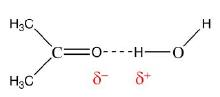The system hydrochloric acid - water has a large enough negative deviation to form a minimum in the vapor pressure curve known as a (negative) azeotrope, corresponding to a mixture that evaporates without change of composition. When these two components are mixed, the reaction is exothermic as ionic bonds H3O+— Cl– are formed so that ΔHmix is negative.

Offer running on EduRev: Apply code STAYHOME200 to get INR 200 off on our premium plan EduRev Infinity!

## Physical Chemistry

66 videos|94 docs|32 tests

,

,

,

,

,

,

,

,

,

,

,

,

,

,

,

,

,

,

,

,

,

;